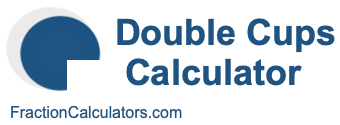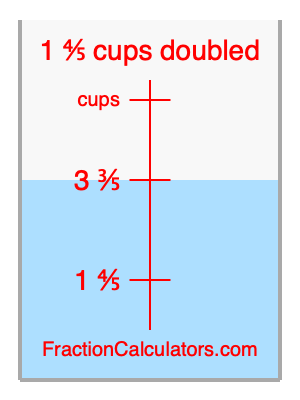What is 1 4/5 cups doubled?Here we will calculate 1 4/5 cups doubled and illustrate 1 4/5 cups doubled on a measuring cup. What is 1 4/5 cups doubled? 1 4/5 cups doubled is twice 1 4/5 cups. Therefore, to double 1 4/5 cups, we multiply 1 4/5 by 2. Here is the formula, the math, and the answer to 1 4/5 cups doubled:

Cup × 2 = Cup doubled
1 4/5 × 2 = 3 3/5
1 4/5 cups doubled = 3 3/5 cups

Below is an illustration of a measuring cup. We show 1 4/5 cups and 1 4/5 cups doubled (3 3/5 cups) so you can see where they are in relation to each other.As you can see, we filled up our measuring cup to 1 4/5 cups doubled. In other words, we filled it up to 3 3/5, which is two times 1 4/5.

Double Cups Calculator
Here you can double another cup measurement.

What is cups doubled?

What is 1 5/6 doubled?
Here is the next measurement on our list that we have doubled for you.